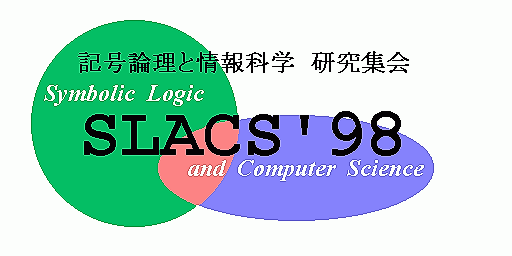15th meeting on Synbolic Logic And Computger Sciences (SLACS'98)

14 -- 16 Oct. 1998
Kawai Hall, Math. Inst., Tohoku Univ.,
Sendai, JAPAN

Invited Talks

Stanley S. Wainer (University of Leeds, UK)

Tilte: Proof Theory and Complexity

This talk will describe some joint work with my student
G. Ostrin, on the proof theory of low subrecursive classes.
The basis is "A new recursion theoretic characterization of
the polytime functions" by Bellantoni and Cook (1992), in
which it is shown that a natural two-sorted re-interpretation
of the normal primitive recursion schemes characterizes
polynomially bounded computation. We show that if Peano
Arithmetic is instead formulated in this two-sorted fashion,
with quantification allowed only over one sort (the "safe"
variables) and induction allowed only over the other ("normal"
variables), then the provably computable functions are exactly
the Kalmar elementary functions E(3). The provably computable
functions of the Sigma_n Inductive fragments of the theory
turn out to be closely related to the levels of Ritchie's
hierarchy of "Predictably computable functions" (1963), with
Sigma_1 Induction corresponding to the linear space functions
E(2). This work is related to other results of Buss, Bellantoni,
Beckmann and Leivant, but in addition it clearly illustrates
the use of classical ordinal analysis techniques at this low level.
The difference with classical Peano Arithmetic is that it is now
the Slow Growing Hierarchy which supplies the bounding functions,
rather than the Fast Growing Hierarchy which arises in the classical
case.

Andreas Weiermann (Westfaelische Wilhelms-Universitaet Muenster, Deutschland)

Title: Sharp bounds for the lengths of reduction sequences in Goedel's T

Abstract: Using ideas from infinitary proof theory and based on Howard's
weak normalization proof for Goedel's system T we define
a numbertheoretic function f such that for two given terms
a and b of T the following holds:
If a rewrites to b then f(a) is strictly larger than f(b).
f ensures termination of T and, in addition,
it yields optimal upper bounds for the lengths of
reductions in Goedel's T.

Jananese Page
Organiser: slacs-kanji@sato.kuis.kyoto-u.ac.jp